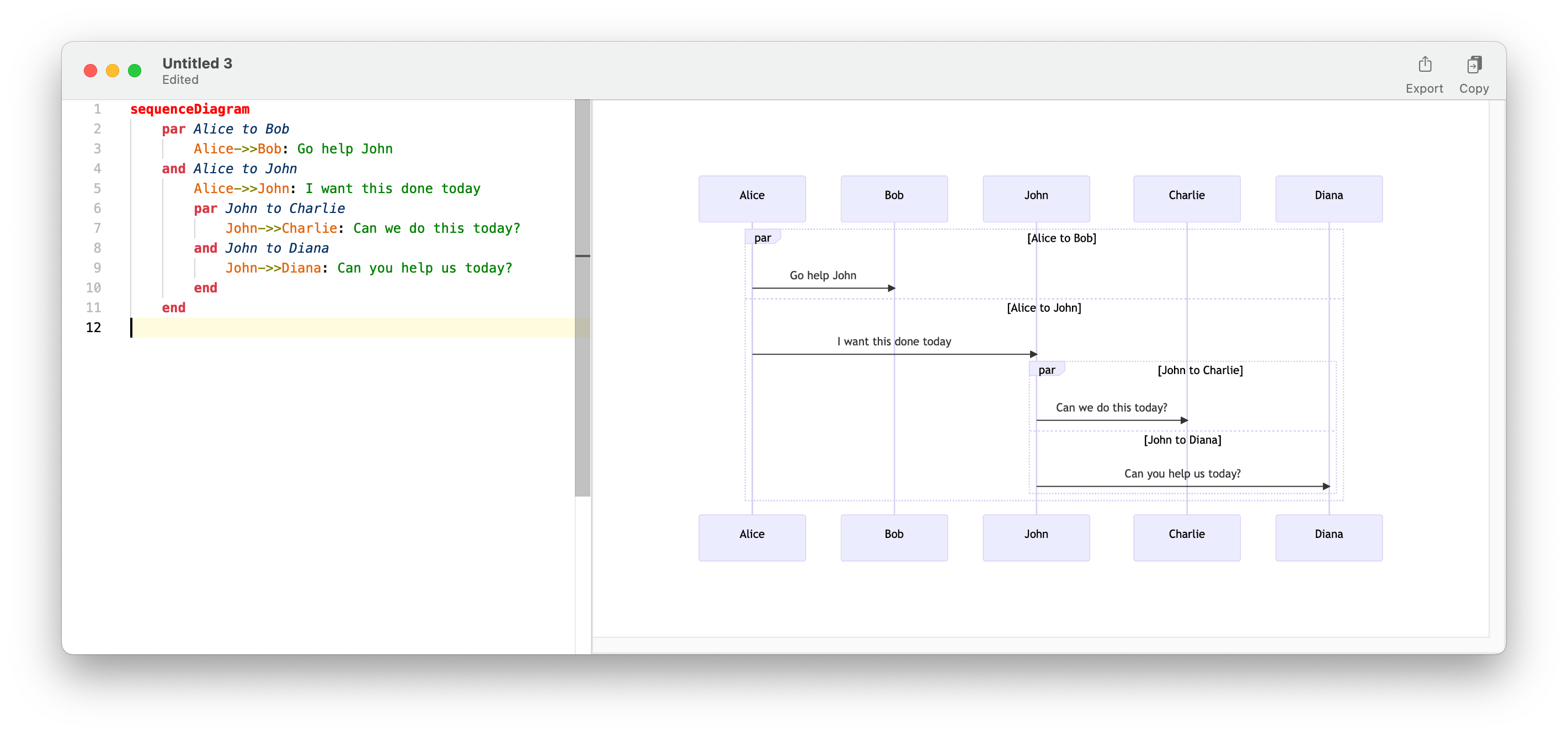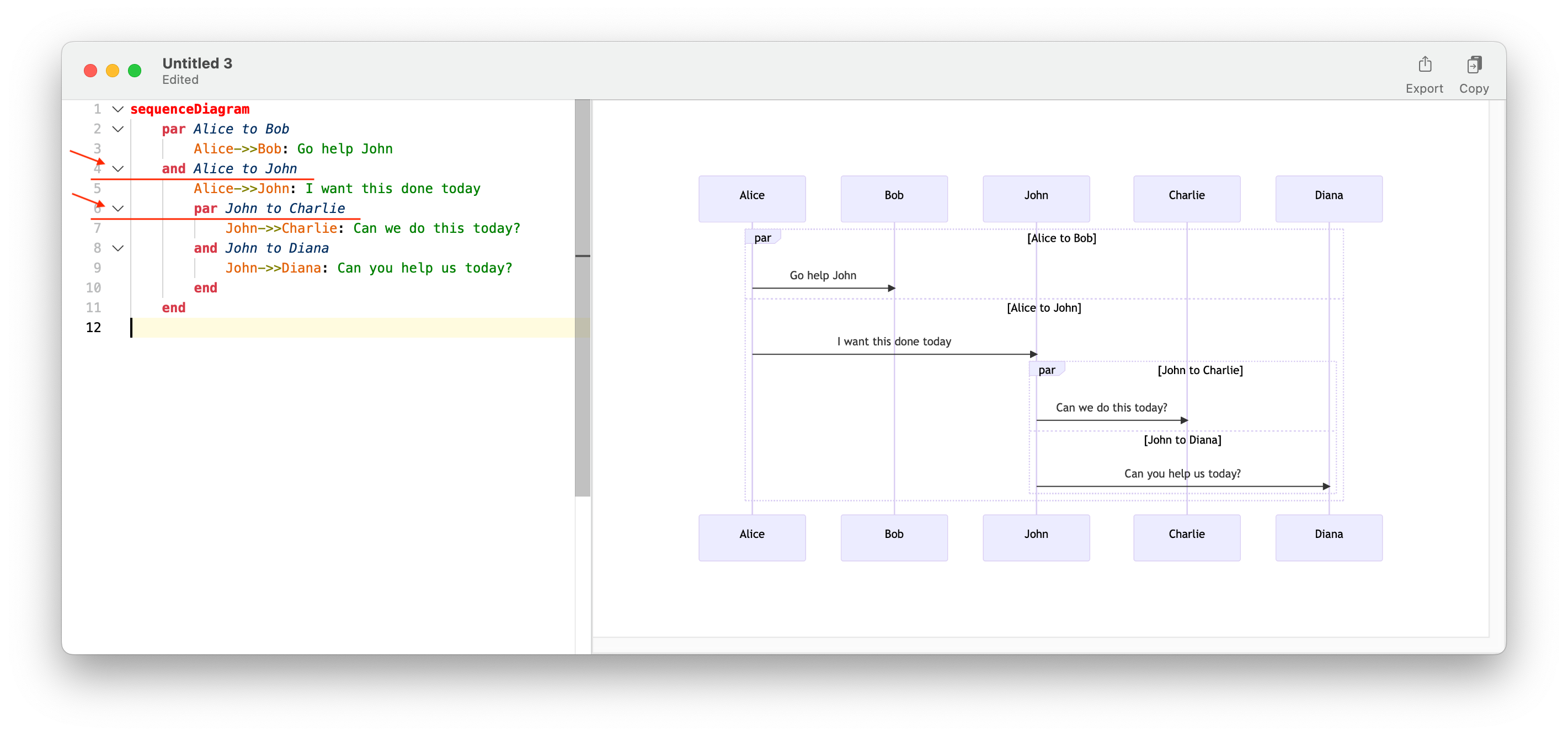## 官方示例

``````monaco.languages.registerFoldingRangeProvider("foldLanguage", {
provideFoldingRanges: function (model, context, token) {
return [
// comment1
{
start: 5,
end: 7,
kind: monaco.languages.FoldingRangeKind.Comment,
},
// ...
]
}
});``````

## 需求定义## 代码实现

``````// 用于匹配每一行空白字符的正则表达式
const pattern = /^(\s*)(.+)/;
// 遍历编辑区内容中的每一行代码
for (var i = 1, count = model.getLineCount(); i <= count; i++) {
const line = model.getLineContent(i);

// 获取每一行代码中的前置空白字符
const matches = pattern.exec(line);
if (matches) {
// 获取当前行的空白长度，用于匹配后续代码行是否属于这一区块
const indentLen = matches.length;
}
}``````

``````// 从下一行开始搜索
let endLine = i + 1;
let lastNotEmptyLine = i;
// 遍历到编辑区内容末尾
while (endLine <= count) {
const lineContent = model.getLineContent(endLine);
// 获取后续行的前置空白字符长度
const subMatches = pattern.exec(lineContent);
if (subMatches != null) {
// 如果前置空白字符长度大于起始行，就将它包含进折叠区域
if (subMatches.length > indentLen) {
lastNotEmptyLine = endLine;
} else {
break;
}
}
endLine++;
}``````

``````if (lastNotEmptyLine > i) {
ranges.push({
start: i,
end: lastNotEmptyLine,
kind: monaco.languages.FoldingRangeKind.Region,
});
}``````

## 完整代码

``````monacoEditor.languages.registerFoldingRangeProvider("foldLanguage", {
provideFoldingRanges: function (model, context, token) {
const ranges = [];
const pattern = /^(\s*)(.+)/;
for (var i = 1, count = model.getLineCount(); i <= count; i++) {
const line = model.getLineContent(i);

const matches = pattern.exec(line);
if (matches) {
const indentLen = matches.length;

let endLine = i + 1;
let lastNotEmptyLine = i;
while (endLine <= count) {
const lineContent = model.getLineContent(endLine);
const subMatches = pattern.exec(lineContent);
if (subMatches != null) {
if (subMatches.length > indentLen) {
lastNotEmptyLine = endLine;
} else {
break;
}
}
endLine++;
}

if (lastNotEmptyLine > i) {
ranges.push({
start: i,
end: lastNotEmptyLine,
kind: monaco.languages.FoldingRangeKind.Region,
});
}
}
}
return ranges;
},
});``````

## 最终效果